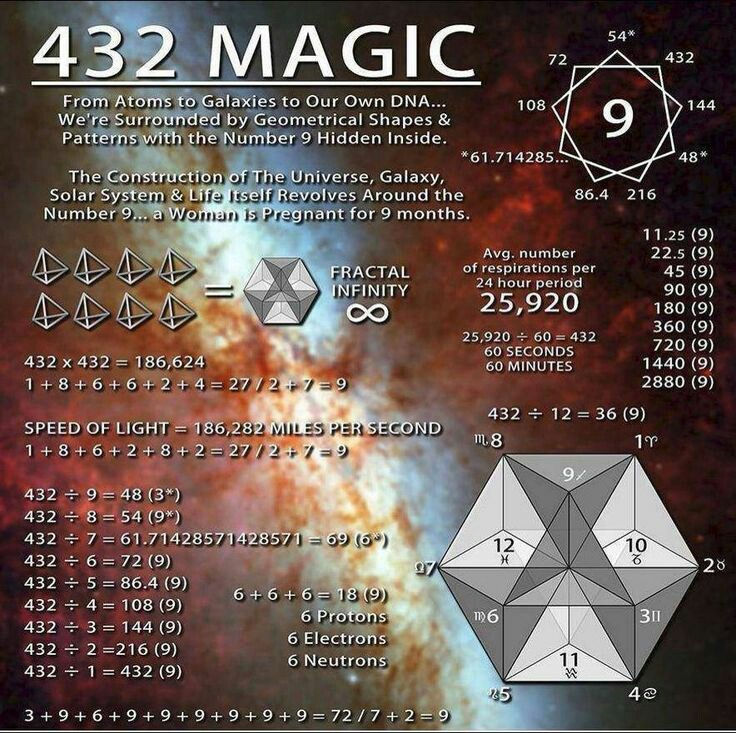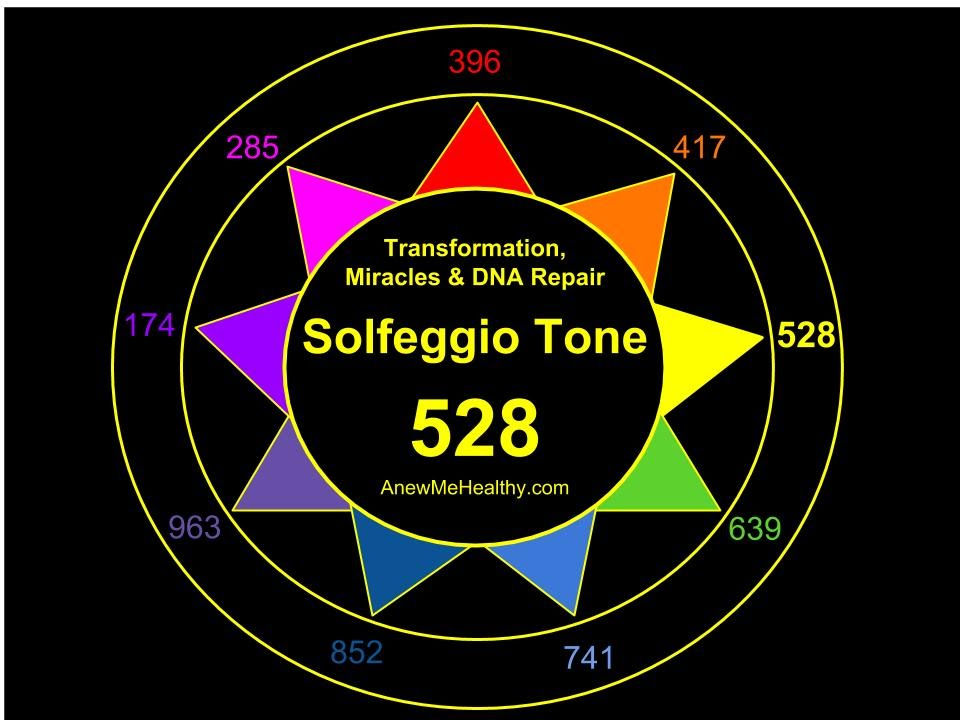top of page
Search

# Infinite tetrahedron grid,infinite dimensional E8 lie group fractal superstring,64 tetrahedron grid,

132+132+132+132=528

528+432=960

528-432=96

432/528=0.81818181

1+{432/528}=1.81818181

528×1.81818181=960

It has been proven that 432Hz and 528Hz are woven together mathematically. Simple calculations show that they both give key numbers for universal construction:

528/432=~1.2

4320×1.2=5184=72²

4320/1.2=3600

528/6(the number of main solfeggio tones)=88

432/[528/432]=360

It is readily apparent from a simple mathematical analysis that A=444Hz,[c(5)=528Hz] and A=432Hz are harmonically related. The harmony can be proven by simply subtracting 432 from 444. It yields 12=1+2=3 in Pythagorean math. If we take 528 and subtract 444 then we can also get 12 or 3. 528-432=96=15=6

This result is identical to 5+2+8=15=6 these sets of numbers 3,6,9 and 8 are always exclusively represented by these special natural pure tones,their scales, and their harmonics.432=432=9

108+108+108+108=432

Tetragrammaton=72=9

108=36:36:36=9:9:9=27=124875(142857)=enneagram

124875(142857)=27=3³=3cubes=6:6:6=18=(star tetrahedron)

27=9

(64 tetrahedron grid)=(8 star tetrahedrons)

(Fruit of life(Metatrons cube(64 tetrahedron grid)))

(Fruit of life)=(2 Fibonacci spirals)=1.618The 432Hz+1.618 is a superstring kugleblits encoding the infinite tetrahedron grid(infinite tetractys/tetragrammaton) which is the infinite dimensional E8 lie group fractalThe fruit of life encodes the infinite tetrahedron grid and all superstrings meaning it is the singularity and its frequency is -0.08333 and its physical frequency is om(432Hz) which is the source/omnigalactic source and it is the vibration and consciousness of the entire infinite multyvers